# Numerical Solution of Layer Equations

The low-field error-field penetration calculations shown in Figure 7.5 use the analytical approximations (7.16) and (7.17) to determine the real and imaginary components of the layer matching parameter,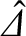. Likewise, the high-field error-field penetration calculations shown in Figure 7.6 use the analytical approximations (7.22) and (7.23) to determine the layer matching parameter. An alternative approach would be to determine the layer matching parameter directly via numerical solution of the layer equations, as described in Section 5.14. The latter approach is more accurate than relying on analytic approximations, but is also much more time consuming.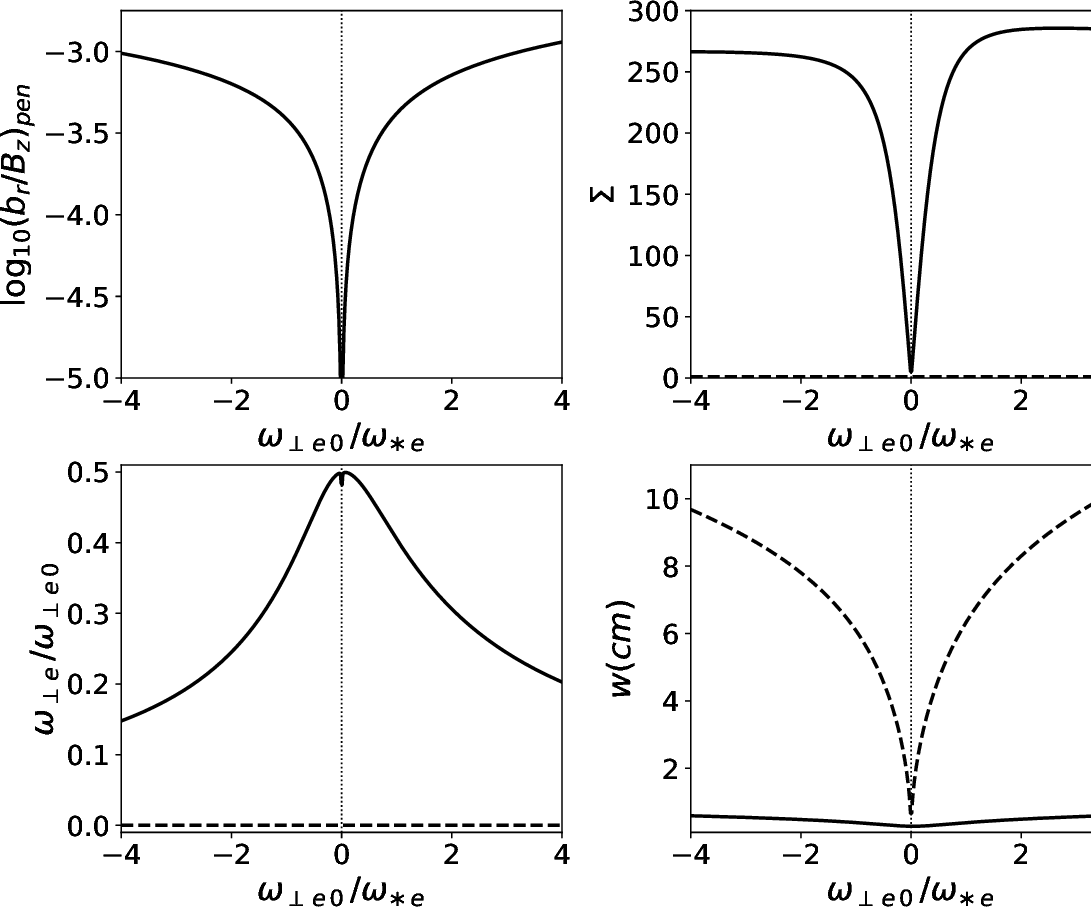Figure 7.7 shows error-field penetration calculations made for a low-field tokamak fusion reactor that employ numerical solutions of the layer equations (with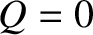, because the error-field is static), rather than analytical approximations, to determine the layer matching parameter. The calculation parameters are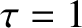,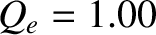,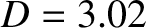,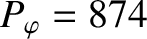, and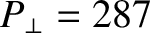, as well as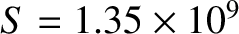,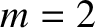, and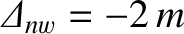, and, finally,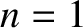,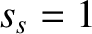,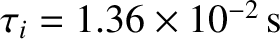,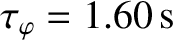,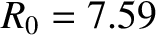m,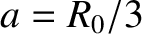, and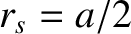. (See Tables 2.1, 5.1, and 6.1.) It can be seen, by comparison with Figure 7.5, that the analytical approximations (7.16) and (7.17) lead to a slight underestimate of the shielding factor prior to penetration. The calculations shown in Figure 7.7 also exhibits a slight asymmetry between positive and negative values of the unperturbed (by the error-field) electron fluid rotation frequency,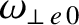, at the rational surface that is not captured by calculations that depend on analytical approximations. (If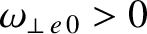then the tearing mode resonant at the rational surface would rotate in the electron diamagnetic direction were it naturally unstable. If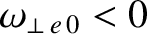then the mode would rotate in the ion diamagnetic direction.) This asymmetry is due to ion diamagnetic flows. Nevertheless, the level of agreement between the calculations shown in Figures 7.5 and 7.7 is sufficiently good to warrant using analytic approximations in calculations that do not require extreme accuracy.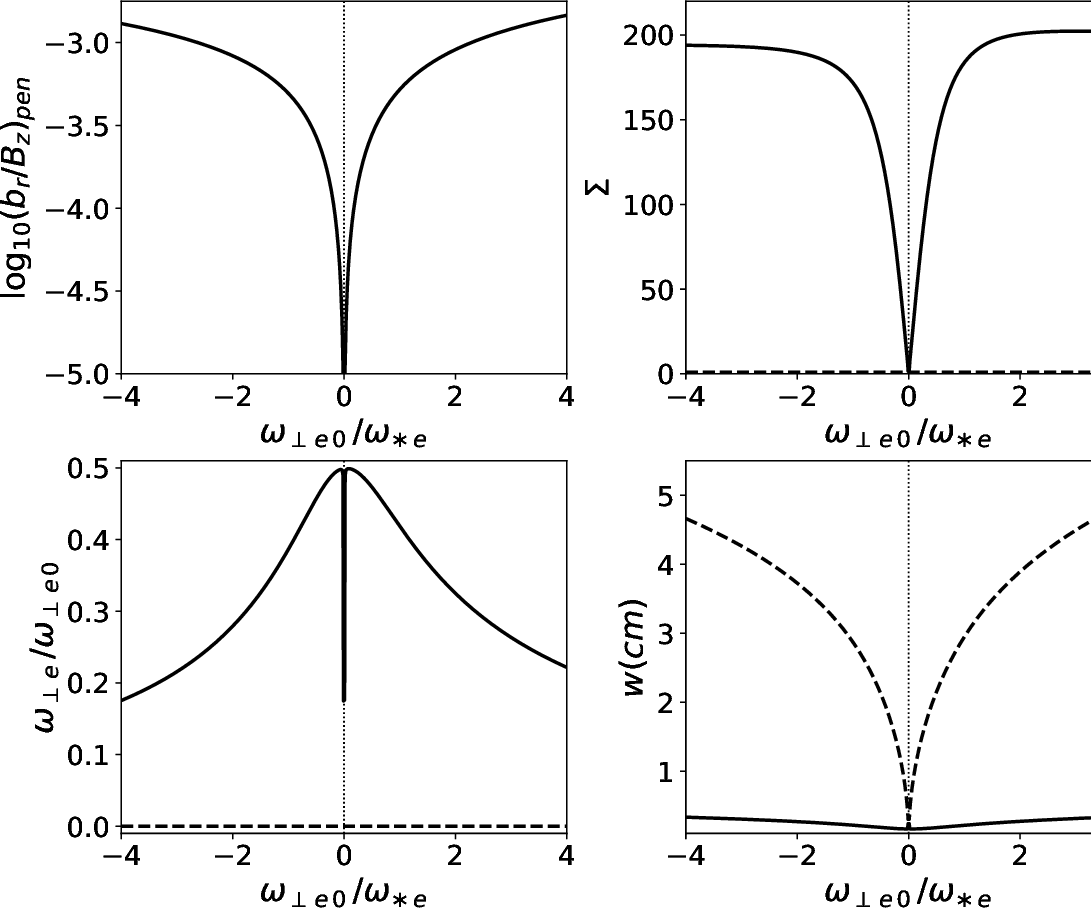Figure 7.8 shows error-field penetration calculations made for a high-field tokamak fusion reactor that employ numerical solutions of the layer equations, rather than analytical approximations, to determine the layer matching parameter. The calculation parameters are,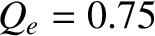,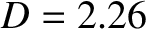,, and, as well as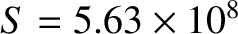,, and, and, finally,,,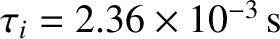,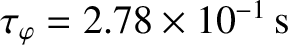,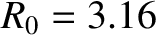m,, and. (See Tables 2.1, 5.1, and 6.1.) Again, it can be seen, by comparison with Figure 7.6, that calculations that rely on analytic approximations lead to a slight underestimate of the shielding factor prior to penetration, and do not capture a slight asymmetry between positive and negative values of the electron fluid rotation frequency at the rational surface.